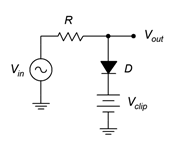# 3.6: Exercises

$$\newcommand{\vecs}{\overset { \rightharpoonup} {\mathbf{#1}} }$$ $$\newcommand{\vecd}{\overset{-\!-\!\rightharpoonup}{\vphantom{a}\smash {#1}}}$$$$\newcommand{\id}{\mathrm{id}}$$ $$\newcommand{\Span}{\mathrm{span}}$$ $$\newcommand{\kernel}{\mathrm{null}\,}$$ $$\newcommand{\range}{\mathrm{range}\,}$$ $$\newcommand{\RealPart}{\mathrm{Re}}$$ $$\newcommand{\ImaginaryPart}{\mathrm{Im}}$$ $$\newcommand{\Argument}{\mathrm{Arg}}$$ $$\newcommand{\norm}{\| #1 \|}$$ $$\newcommand{\inner}{\langle #1, #2 \rangle}$$ $$\newcommand{\Span}{\mathrm{span}}$$ $$\newcommand{\id}{\mathrm{id}}$$ $$\newcommand{\Span}{\mathrm{span}}$$ $$\newcommand{\kernel}{\mathrm{null}\,}$$ $$\newcommand{\range}{\mathrm{range}\,}$$ $$\newcommand{\RealPart}{\mathrm{Re}}$$ $$\newcommand{\ImaginaryPart}{\mathrm{Im}}$$ $$\newcommand{\Argument}{\mathrm{Arg}}$$ $$\newcommand{\norm}{\| #1 \|}$$ $$\newcommand{\inner}{\langle #1, #2 \rangle}$$ $$\newcommand{\Span}{\mathrm{span}}$$$$\newcommand{\AA}{\unicode[.8,0]{x212B}}$$

(Assume diodes are silicon unless stated otherwise)

## 3.6.1: Analysis Problems

1. For the circuit of Figure $$\PageIndex{1}$$, determine the peak output voltage. $$V_{sec} = 12$$ volts RMS, $$R_{load} = 50$$ $$\Omega$$, $$C_1 = 1500$$ $$\mu$$F.Figure $$\PageIndex{1}$$

2. Sketch the output voltage waveform for the circuit of Problem 1, Figure $$\PageIndex{1}$$, with and without the capacitor.

3. Determine the peak output voltage for the circuit of Figure $$\PageIndex{2}$$. $$V_{sec} = 18$$ volts RMS, $$R_{load} = 75$$ $$\Omega$$, $$C_1 = 470$$ $$\mu$$F.Figure $$\PageIndex{2}$$

4. Sketch the output voltage waveform for the circuit of Problem 3, Figure $$\PageIndex{2}$$, with and without the capacitor.

5. For the circuit of Figure $$\PageIndex{3}$$, determine the peak output voltage. $$V_{sec} = 18$$ volts RMS, $$R_{load} = 40$$ $$\Omega$$, $$C_1 = 1000$$ $$\mu$$F.Figure $$\PageIndex{3}$$

6. Sketch the output voltage waveform for the circuit of Problem 5, Figure $$\PageIndex{3}$$, with and without the capacitor.

7. Determine the output voltage waveform and its amplitude for the circuit of Figure $$\PageIndex{4}$$. $$V_{in} = 10 \sin 2\pi 100t$$, $$V_{clip} = 8$$ volts, $$R = 10$$ k$$\Omega$$.Figure $$\PageIndex{4}$$

8. Draw the output waveform with its amplitudes for the circuit of Figure $$\PageIndex{5}$$. $$V_{in} = 10 \sin 2\pi 100t$$, $$V_{clip} = 5$$ volts, $$R = 10$$ k$$\Omega$$.Figure $$\PageIndex{5}$$

9. Draw the output waveform with its amplitudes for the circuit of Figure $$\PageIndex{6}$$. $$V_{in} = 12 \sin 2\pi 200t$$, $$V_1 = 6$$ volts, $$V_2 = 4$$ volts, $$R = 10$$ k$$\Omega$$.Figure $$\PageIndex{6}$$

10. Draw the output waveform with its amplitudes for the circuit of Figure $$\PageIndex{7}$$. $$V_{in} = 5 \sin 2\pi 2000t$$, $$C = 10$$ $$\mu$$F, $$R = 4.7$$ k$$\Omega$$.Figure $$\PageIndex{7}$$

11. Draw the output waveform with its amplitudes for the circuit of Figure $$\PageIndex{8}$$. $$V_{in} = 8 \sin 2\pi 500t$$, $$V_{clamp} = 2$$ volts, $$C = 4.7$$ $$\mu$$F, $$R = 33$$ k$$\Omega$$.Figure $$\PageIndex{8}$$

## 3.6.2: Design Problems

12. Design a 15 volt AC to DC power supply capable of drawing 200 mA.

13. Design a circuit that will limit its output voltage to a range of −5 volts to +10 volts.

14. Design a circuit that will shift its output voltage so that it is always positive. The input frequency is 2 kHz.

## 3.6.3: Challenge Problems

15. Design a circuit that will shift its output voltage so that its negative peak is at +3 volts. The input frequency range is from 100 Hz to 1 kHz.

## 3.6.4: Computer Simulation Problems

16. Run a transient analysis of the circuit in Figure $$\PageIndex{1}$$, Problem 1.

17. Run a transient analysis of the circuit in Figure $$\PageIndex{2}$$, Problem 3.

18. Run a transient analysis of the circuit in Figure $$\PageIndex{3}$$, Problem 5.

19. Run two transient analyses on the clamper circuit of Example 3.4.1, first using a capacitor 100 times larger than specified, and second using a capacitor 100 times smaller than specified. Discuss the resulting waveforms.

This page titled 3.6: Exercises is shared under a CC BY-NC-SA 4.0 license and was authored, remixed, and/or curated by James M. Fiore via source content that was edited to the style and standards of the LibreTexts platform; a detailed edit history is available upon request.